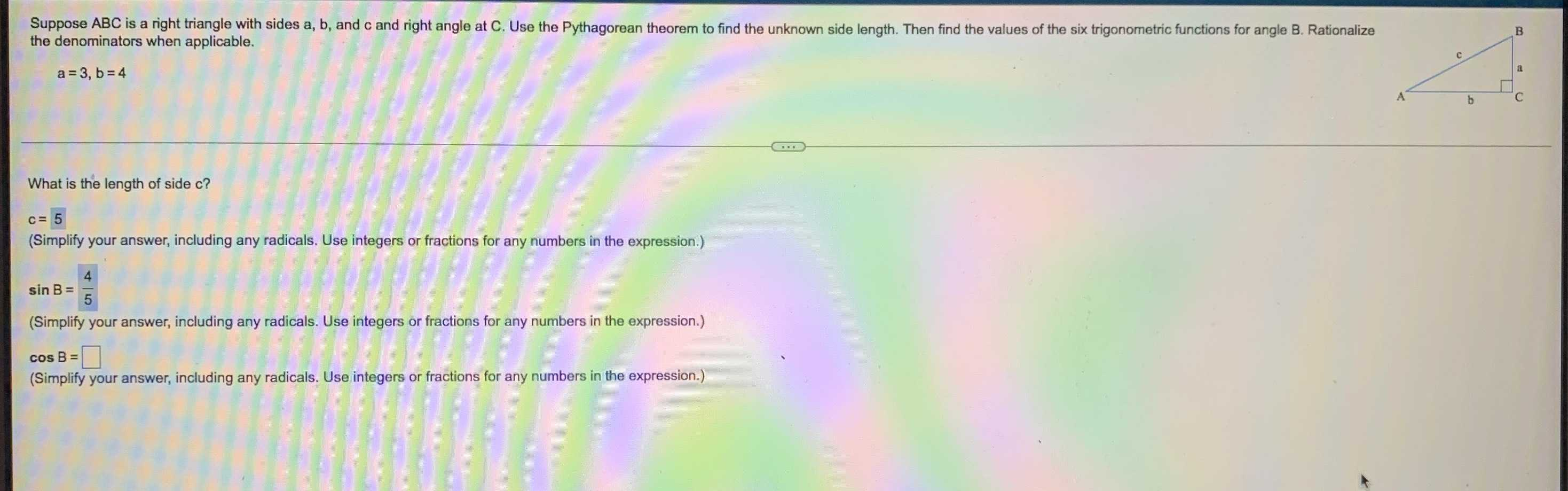### Still have math questions?

Trigonometry
QuestionSuppose $$A B C$$ is a right triangle with sides a, b, and c and right angle at C. Use the Pythagorean theorem to find the unknown s the denominators when apolicable. nide length. Then find the values of the six trigonometric functions for angle B. Rationalize the denominators when applicable. $$a = 3 , b = 4$$

What is the length of side c? (Simplify your answer, including any radicals. Use integers or fractions for any numbers in the expression.)

$$\sin B = ?$$

$$\sin B = \frac { 4} { 5} \\ \cos B = \frac { 3} { 5} \\ \tan B = \frac { 4} { 3} \\ \cos ec B = \frac { 5} { 4} \\ \sec B = \frac { 5} { 3} \\ \cot B = \frac { 3} { 4}$$